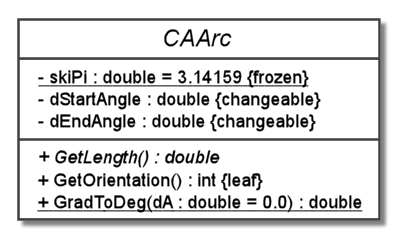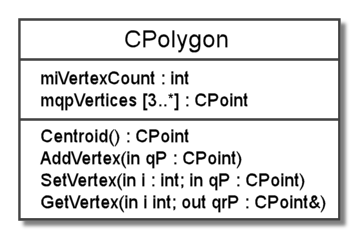# Bits & Bytes

## Posts Tagged ‘diagram’

### UML Class Diagrams – part 4

Continued . . .

In this section, we give the final details of the attributes and operations that can be modeled with UML. With the central elements covered, we are going to add some examples of attributes with initial values and operations with default argument values. Additionally, we will give some examples of attributes and operations with properties, and we will talk about some of the commonly used properties. Finally, we will show how to of model scope and operation binding.

Our arc class below, called CAArc, should serve to illustrate the remaining elements of UML that can be modeled for operations and attributes. This class contains three operations and three attributes. Of the operations, two are instance operations and the third is a class operation. The word static is used in C++ to indicate a class member, rather than an instance member. The word virtual before GetLength() indicates that that function has dynamic binding, while GetOrientation() is statically, or compile-time, bound. Also, the ” = 0″ is used to indicate that GetLength() is not implemented for this class, but rather is a pure virtual function or an abstract operation.

Of the classes attributes, two are instance members and one, the constant skdPi, is a class member. The constant is initialized at compile-time to the value 3.14159. The other member variables, dStartAngle and dEndAngle, have no initial value that is apparent here and are non-constant, so they can be changed at anytime.

```class CAArc
{
public:
virtual double GetLength() = 0;
int GetOrientation();
static double GradToDeg(double dA = 0.0);
private:
static const double skdPi = 3.14159;
double dStartAngle;
double dEndAngle;
};```

The arc class above can be modeled in UML using the diagram below. Since the GetLength() is an abstract operation, we italicize it to show this. We use the property “leaf” to indicate that the operation GetOrientation() is statically bound and can not be overridden. The operation is GradToDeg() has an argument with a default argument value, as shown. Also, we have underlined GradToDeg() to show that it is a class operation. Likewise, we underline skdPi, since it is a constant with class scope. The initial value for skdPi is shown along with the qualifier “frozen” to indicate that this is a constant. The instance members dStartAngle and dEndAngle are just ordinary member variables.As far as properties, notice that the constant skiPi has the property “frozen” after it and the function GetOrientation() has the property “leaf” after it. However, we also have the property “changeable” on our instance variables. This can be used with attributes to specify that the variable’s value can be changed. We can also use “addOnly” on attributes with multiplicity to indicate that the values can only be added, say to an array, for instance. For operations, we can use the property “isQuery” to indicate that the operation does not change values; this is the C++ equivalent of a function that is constant. Additionally, we can specify operations having the properties “sequential”, “guarded”, or “concurrent” in the context of processes and threads.

To be continued . . .

### UML Class Diagrams – part 2

Continued . . .

Previously, we demonstrated a basic class diagram of a simple class that could be used for creating points in the plane. Now we will expand on the elements of what we showed before with some additional details in our UML class diagram. To be sure, we will still have more details to add when we are done here, but rest assured we will fill out everything in the end.

We begin with the C++ header code below for a polygon class, called CPolygon; this class could be used in conjunction with our previous point class to represent polygons in the plane. This polygon class consists of two member variables: an int to hold a count of the number of vertices in the polygon and a pointer to a dynamically allocated array of vertices that are CPoint objects. Additionally, there are four simple member functions to calculate the centroid (center point), add a vertex, set a vertex, and get a vertex. For simplicity, we have ignored constructors and the destructor.

```class CPolygon
{
public:
CPoint Centroid();
void SetVertex(int i, CPoint qP);
void GetVertex(int i, CPoint& qrP);

private:
int miVertexCount;
CPoint* mqpVertices;
};```

A UML diagram for this class is shown below. Notice that this diagram includes some details that we did not show previously. We have added the member variable types, which we tacked on with a colon after the variable names. Also, directly after the variable name mqpVertices, we have added [3..*] to represent the multiplicity of the vertices in our polygon (a polygon has three or more vertices). Presumbaly, the vertices array would be reallocated and copied when AddVertex() is called to add a vertex or something like that, but we have not specified the exact behavior here.For our operations, we add the return type after a colon. This is evident with the Centroid() function, since it has a return type of CPoint. The remaining functions have a void return type, so they return nothing. We could have shown a void return type, but we would probably never bother in actual practice. Likewise, we have omitted the void argument type for the Centroid() function. Unlike the return type, we can omit this, as we did, in the C++ code as well.

For the last three operations, we have member functions that take arguments. Since the AddVertex() function takes only one argument, we will start with it. Like our member variables, arguments have a name, then a colon, and finally the type of the argument. However, in AddVertex(), this is all preceded by the word “in” that is used to indicate that this is an input argument.

In this context, input means that the value that is passed in is important. In the GetVertex() function, the second argument is preceded by the word “out” to indicate that it is and output argument. In this case, the value that is passed in is not important, but the value that the argument variable has after the call is important. If both the input and output values are important, than we use “inout” to signify this. Lastly, notice that we replaced the commas that separated functional arguments in the C++ code with semicolons.

To be continued . . .